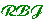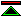# Classical Greek Mathematics

 During the period from about 600 B.C. to 300 B.C. , known as the classical period of Greek mathematics, mathematics was transformed from an ecclectic collection of practical techniques into a coherent structure of deductive knowledge. For many mathematicians, the discipline we call mathematics was founded in this period. Here we briefly survey the achievements from a logical point of view.

 From Procedural to Declarative Knowledge The change of focus from practical problem solving methods to knowledge of general mathematical truths and the development of a body of theory transforms mathematics into a scientific discipline. Abstraction Pythagorean abstraction and Plato's "ideals" make the subject matter of mathematics out of this world. Logic The cannons of deductive reasoning are systematised by Aristotle in his syllogistic logic. Foundations The Greeks showed concern for the logical structure of mathematics. The Pythagorean's sought to found all of mathematics on number but were confounded by the discovery of incommensurable ratios in geometry. This prevented them from giving an account of geometric magnitudes in terms of their numbers (what we now call the natural numbers or positive integers). By the end of the Pythagorean period geometry has come to be regarded as fundamental. The problem of incommensurable ratios will remain unresolved for more than two millenia. Deduction From very early in the classical period deduction is perceived as the primary method of arriving at mathematical truths. This contrasts with (but does not entirely displace) non-deductive generalisation from particulars. Proof By the end of the classical period the requirement that mathematical results should be subject to proof has been firmly established. The Axiomatic Method In Euclid's Elements, the most influential mathematical text in history, we see established the axiomatic method. This enables Euclid to organize deductive proofs into a systematic, rigorous and well organized presentation of a substantial body of mathematical knowledge. Definition, Consistency and Existence. Within the axiomatic method, definitions have their place. Aristotle has pointed out the need in definitions for the definiendum to exist, and in Euclid's geometry this is achieved by requiring all geometric figures to be constructible by ruler and compass. Thus we see first a concern for consistency, and then a systematic response to that concern.©created 96/8/10 modified 96/10/27